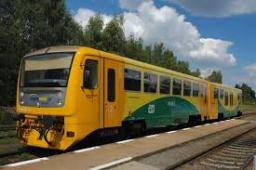# Distance 3295

If the train travels at an average speed of 60 km/h, it will cover a certain distance in 5 hours and 30 minutes. What is the average speed he travels to cover the same distance in 5 hours?

v =  66 km/h

### Step-by-step explanation:

60·(5+30/60)=v·5

300v = 19800

v = 19800/300 = 66

v = 66

Our simple equation calculator calculates it.Did you find an error or inaccuracy? Feel free to write us. Thank you!

Tips for related online calculators
Do you want to convert length units?
Do you want to convert velocity (speed) units?
Do you want to convert time units like minutes to seconds?